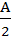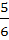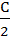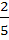Question 112

In a triangle tan=, tan=, then

a) a, c, b are in Arithmetic Progression

b) a, b, c are in Arithmetic Progression

c) b, a, c are in Arithmetic Progression

d) a, b, c are in Geometric Progression

AIEEE 2002